Courses

# NCERT Solutions - Atoms And Molecules Class 9 Notes | EduRev

## UPSC : NCERT Solutions - Atoms And Molecules Class 9 Notes | EduRev

The document NCERT Solutions - Atoms And Molecules Class 9 Notes | EduRev is a part of the UPSC Course NCERT Textbooks (Class 6 to Class 12).
All you need of UPSC at this link: UPSC

Page No. 32

Q.1. In a reaction, 5.3 g of sodium carbonate reacted with 6 g of ethanoic acid. The products were 2.2 g of carbon dioxide, 0.9 g water and 8.2 g of sodium ethanoate. Show that these observations are in agreement with the law of conservation of mass.
Sodium carbonate ethanoic acid → sodium ethanoate carbon dioxide water
Ans.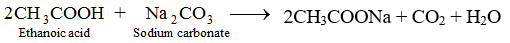► Mass of reactants = 5.3 g + 6 g = 11.3 g
► Mass of products = 2.2 g + 0.9 g + 8.2 g = 11.3 g
► Mass of reactants = Mass of products
Therefore, the Law of conservation of mass is proved.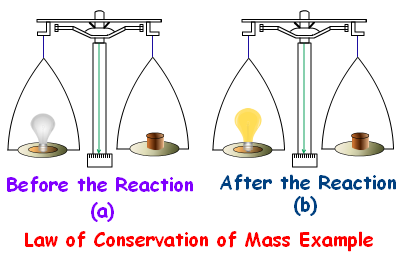Q.2. Hydrogen and oxygen combine in the ratio of 1:8 by mass to form water. What mass of oxygen gas would be required to react completely with 3g of hydrogen gas?
Ans. Since hydrogen and oxygen combine in the ratio of 1:8 by mass, 3g of hydrogen gas will react completely with 24 g of oxygen gas.

Q.3. Which postulate of Dalton's atomic theory is the result of the law of conservation of mass?
Ans. Dalton’s postulate that “Atoms can neither be created nor destroyed,” is a result of law of conservation of mass.

Q.4. Which postulate of Dalton's atomic theory can explain the law of definite proportions?
Ans. Atoms combine in fixed ratio to form compounds, can explain the law of definite proportions.

Page No. 35

Q.1. Define atomic mass unit.
Ans. It is defined as equal 1/12th of mass of 1 atom of C-12. It is called unified mass denoted by 'u’ these days.

Q.2. Why is it not possible to see an atom with naked eyes?
Ans. The size of an atom is so small that it is not possible to see it with naked eyes. Also, the atom of an element does not exist independently.

Page No. 39

Q.1. Write down the formulae of
(a) sodium oxide
(b) aluminium chloride
(c) sodium sulphide
(d) magnesium hydroxide
Ans.
(a) Formula of Sodium Oxide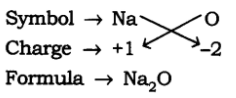(b) Formula of Aluminium Chloride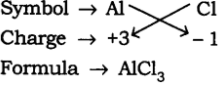(c) Formula of Sodium Sulphide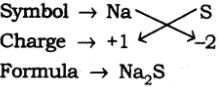(d) Formula of Magnesium Hydroxide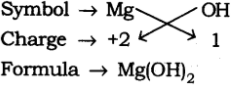Q.2. Write down the names of compounds represented by the following formulae:
(a) Al2(SO4)3

(b) CaCl2
(c) K2SO4
(d) KNO3
(e) CaCO3
Ans.
(a) Aluminium sulphate
(b) Calcium chloride
(c) Potassium sulphate
(d) Potassium nitrate
(e) Calcium carbonate

Q.3. What is meant by the term chemical formula?
Ans. The chemical formula of a compound is a symbolic representation of its composition.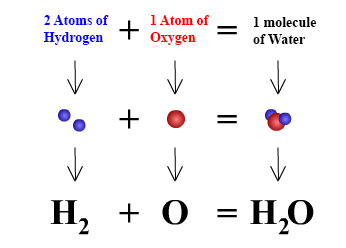Chemical formula of waterQ.4
. How many atoms are present in a
(a) H2S molecule and
(b) PO43- ion?

Ans.
(a) 3 atoms are present is H2S.
(b) 5 atoms are present in PO43- ion

Page No. 40

Q.1. Calculate the molecular masses of H2, O2, Cl2, CO2, CH4, C2H6, C2H4, NH3, CH3OH.
Ans.

► Molecular mass of H= 2 × Atomic mass of H
= 2 × 1
= 2 u
► Molecular mass of O= 2 × Atomic mass of O
= 2 × 16
= 32 u
► Molecular mass of Cl= 2 × Atomic mass of Cl
= 2 × 35.5
= 71 u
► Molecular mass of CO= Atomic mass of C2 × Atomic mass of O
= 12+ (2+16) = (12 + 32)u
= 44 u
► Molecular mass of CH4= Atomic mass of C4 × Atomic mass of H
= 12+ (4 x 1)u = (12 + 4)u
= 16 u
► Molecular mass of C2H= 2× Atomic mass of C6× Atomic mass of H
= (2 x 12 + 6 x 1)u = (24 + 6)u
= 30 u
► Molecular mass of C2H= 2 x Atomic mass of C4 × Atomic mass of H
= (2 x 12 + 4 x 1)u = (24 + 4)u
= 28 u
► Molecular mass of NH= Atomic mass of N3 × Atomic mass of H
= (14 + 3 x 1)u = (14 + 3)u
= 17 u
► Molecular mass of CH3OH = Atomic mass of C3 × Atomic mass of H Atomic mass of O Atomic mass of H
= (12 + 3 x 1 + 16 + 1)u = (12 + 3 + 17)u
= 32 u

Q.2. Calculate the formula unit masses of ZnO, Na2O, K2CO3, given atomic masses of Zn = 65 u, Na = 23 u, K = 39 u, C = 12 u, and O = 16 u.
Ans.

(i) Formula unit mass of ZnO
= 65 + 16 = 81 u
(ii) Formula unit mass of Na2O
= 2 x 23 + 16 = 46 + 16 = 62 u
(iii) Formula unit mass of K2CO3
= 2 x 39 + 12 + 3 x 16
= 78 + 12 + 48 = 138 u

Page No. 42

Q.1. If one mole of carbon atoms weighs 12 gram, what is the mass (in gram) of 1 atom of carbon?
Ans.

► 1 mole of carbon atoms = 12 g
► 1 mole of carbon contains = 6.022 x 1023 atoms
► 6.022 x 1023 atoms weigh = 12 g
► 1 atom of carbon weighs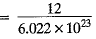= 1.99 x 10-23 g

Q.2. Which has more number of atoms, 100 grams of sodium or 100 grams of iron (given, atomic mass of Na = 23 u, Fe = 56 u)?
Ans.

► No. of moles of Na = 100/23 = 4.34 moles
► No. of moles of Fe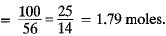Since the number of moles of Na is more than the number of moles of Fe, therefore, 100 g of Na contains more number of atoms.

Page No. 43

Q.1. A 0.24 g sample of compound of oxygen and boron was found by analysis to contain 0.096 g of boron and 0.144 g of oxygen.
Calculate the percentage composition of the compound by weight.
Ans.

► Percentage of boron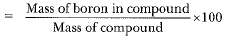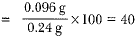► Percentage of oxygen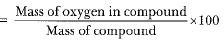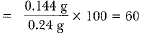Q.2. When 3.0 g of carbon is burnt in 8.00 g oxygen, 11.00 g of carbon dioxide is produced. What mass of carbon dioxide will be formed when 3.00 g of carbon is burnt in 50.00 g of oxygen? Which law of chemical combinations will govern your answer?
Ans.

► C + O2 → CO2
► 12 g of C reacts with 32 g of O2
► 3 g of C reacts with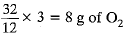► 3 g of carbon + 8 g of oxygen = 11 g of Carbon dioxide
► 3 g of carbon will react with only 8.0 g of oxygen to form 11 g of carbon dioxide.
► 50 - 8 = 42 g of O2 will remain unreacted.
The law of constant proportion will govern answer because carbon and oxygen combine in a fixed ratio.

Q.3. What are polyatomic ions? Give examples?
Ans. The ion which is made up of more than one atom is called a polyatomic ion.
Example: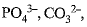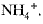Q.4. Write the chemical formulae of the following:
(a) Magnesium chloride

(b) Calcium oxide
(c) Copper nitrate
(d) Aluminium chloride
(e) Calcium carbonate.
Ans.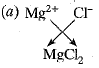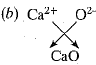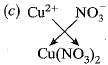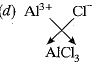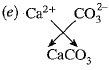Q.5. Give the names of the elements present in the following compounds:
(a) Quick lime

(b) Hydrogen bromide
(c) Baking powder
(d) Potassium sulphate
Ans.

(a) Quick lime contains calcium and oxygen.
(b) Hydrogen bromide contains hydrogen and bromine.
(c) Baking powder (NaHCO3) contains sodium, hydrogen, carbon and oxygen.
(d) Potassium sulphate contains potassium, sulphur and oxygen.

Q.6. Calculate the molar mass of the following substances:
(a) Ethyne, C2H2

(b) Sulphur molecule, S8
(c) Phosphorus molecule, P4 (atomic mass of phosphorus = 31)
(d) Hydrochloric acid, HCl
(e) Nitric acid, HNO3
Ans.
(a) Molar mass of ethyne (C2H2) = 2 x 12 + 1 x 2 = 24 + 2 = 26 g
(b) Molar mass of sulphur molecule (S8) = 8 x 32 = 256 g
(c) Molar mass of phosphorus molecule (P4) = 4 x 31 = 124 g
(d) Molar mass of hydrochloric acid (HCI) = 1 + 35.5 = 36.5 g
(e) Molar mass of nitric acid (HNO3= 1 + 14 + 3 x 16 = 1 + 14 + 48 = 63 g

Q.7. What is the mass of
(a) 1 mole of nitrogen atoms?

(b) 4 moles of aluminium atoms (Atomic mass of aluminium = 27)?
(c) 10 moles of sodium sulphite (Na2SO3)?
Ans.
(a) Mass of 1 mole of nitrogen atoms = 14 g
(b) Mass of 1 mole of aluminium atoms = 27 g
Mass of 4 moles of aluminium atoms = 27 x 4 = 108 g
(c) Mass of 1 mole of Na2SO= 2 x 23 + 32 x 1 + 3 x 16

= 46 + 32 + 48 = 126 g
Mass of 10 moles of Na2SO3 = 126 x 10 = 1260 g

Q.8. Convert into mole.
(a) 12 g of oxygen gas
(b) 20 g of water
(c) 22 g of carbon dioxide

Ans.

(a) 1 mole oxygen gas = 32 g
Number of moles of oxygen gas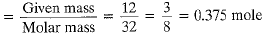(b)  1 mole of H2O = 2 + 16 = 18 g
Number of moles of H2O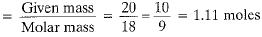(c) 1 mole of CO2 = 12 + 2 x 16 = 44 g
Number of moles of CO2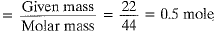Q.9. What is the mass of:
(a) 0.2 mole of oxygen atoms?
(b) 0.5 mole of water molecules?

Ans.

(a) Mass of one mole of oxygen atoms = 16 g
Then, mass of 0.2 mole of oxygen atoms = 0.2 × 16g = 3.2 g
(b) Mass of 1 mole of H2O molecules

= 2 + 16 = 18 g
Mass of 0.5 mole of H2O molecules
= 0.5 x 18 g = 9 g

Q.10. Calculate the number of molecules of sulphur (S8) present in 16 g of solid sulphur.
Ans.

► Number of moles of sulphur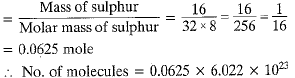= 3.76 x 1023

Q.11. Calculate the number of aluminium ions present in 0.051 g of aluminium oxide.
(Hint: The mass of an ion is the same as that of an atom of the same element. Atomic mass of Al = 27 u)

Ans.
► Molecular weight of Al2O3 = = 2 x 27 + 3 x 16 = 54 + 48 = 102 g mol-1
► 102 g of Al2O3 contains 2 x 6.022 x 1023 Al3+ions
► 0.051 g of Al2O3 contains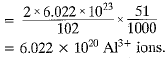Offer running on EduRev: Apply code STAYHOME200 to get INR 200 off on our premium plan EduRev Infinity!

## NCERT Textbooks (Class 6 to Class 12)

954 docs|585 tests

,

,

,

,

,

,

,

,

,

,

,

,

,

,

,

,

,

,

,

,

,

;